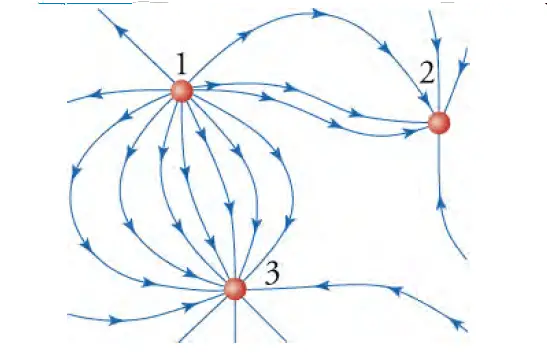# How to determine Relative strength & sign of charges from lines of force

In this post, we will find How to determine the Relative strength & sign of charges from lines of force seen in the drawing or map of the electric field.

## Strategy to determine Relative strength & sign of charges from the drawing of the electric field

We use the following concepts to determine the relative strength and sign of charges:

• We know the electric field extends out from the positive charge and terminates on the negative charge.
• We also know that the number of electric field lines that touch a charge is proportional to the charge.

## Case study – to determine Relative strength & sign of charges from the drawing of the electric field

Let’s consider the electric field shown in figure 1.figure 1: Map of electric field due to three charged particles – [Case study – to determine Relative strength & sign of charges from the drawing of the electric field]

Charge 1 has 12 fields coming out of it. Charge 2 has six field lines going into it. Charge 3 has 12 field lines going into it.

Solution
The electric-field lines come out of charge 1, so it is a positive charge. The electric-field lines go into charges 2 and 3, so they are negative charges.

The ratio of the charges is q1 : q2 : q3 = +12 : -6 : +12. Thus, the magnitude of charges 1 and 3 is twice that of charge 2.
of charge 2.

## Take Away

Although we cannot determine the precise charge on each particle, we can get a lot of information from the electric field regarding the magnitude and sign of the charges and where the force on a test charge would be greatest (or least).